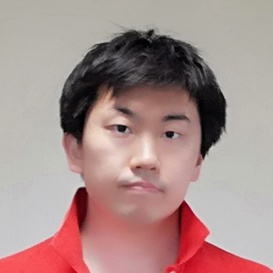AOJ

# # Problem

http://judge.u-aizu.ac.jp/onlinejudge/description.jsp?id=CGL_7_E

# # Explanation

Refer the link below for algorithm.

Algorithm to find cross points of circles

# # Solution

Int N;
double R1, R2;
Vector2 C1, C2;

pair<Vector2, Vector2> crossPoints() {
Vector2 baseVec = C2 - C1;
double baseLen = baseVec.length();
double cos_ = (baseLen*baseLen + R1*R1 - R2*R2) / (2 * baseLen * R1);
double sin_ = sqrt(1 - cos_*cos_);
// Counter-clockwise
Vector2 a_(cos_ * baseVec.x + -sin_ * baseVec.y, sin_ * baseVec.x + cos_ * baseVec.y);
// Clockwise
Vector2 b_(cos_ * baseVec.x + sin_ * baseVec.y, -sin_ * baseVec.x + cos_ * baseVec.y);
Vector2 a = C1 + a_ * R1 / baseLen;
Vector2 b = C1 + b_ * R1 / baseLen;
if (fabs(a.x) < EPS) a.x = 0.0;
if (fabs(a.y) < EPS) a.y = 0.0;
if (fabs(b.x) < EPS) b.x = 0.0;
if (fabs(b.y) < EPS) b.y = 0.0;
if (a < b) return make_pair(a, b);
return make_pair(b, a);
}

void solve() {
auto points = crossPoints();
cout << points.first.x << ' ' << points.first.y << ' ';
cout << points.second.x << ' ' << points.second.y << endl;
}

void input() {
cin >> C1 >> R1 >> C2 >> R2;
}

int main() {
cout.precision(15);
input();
solve();
}Remote freelancer. A web and mobile application enginner.
Traveling around the world based on East Asia.
I'm looking forward to your job offers from all over the world!

Offer jobs or contact me!